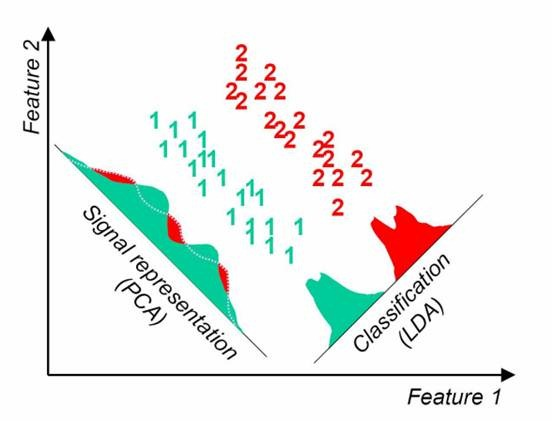# 0-1回归

## 模型构造

### 构造线性概率模型

$y_{i}=\beta_{0}+\beta_{1} x_{1 i}+\beta_{2} x_{2 i}+\cdots+\beta_{k} x_{k i}+\mu_{i}$

㝍成向量乘积形式: $y_{i}=\boldsymbol{x}_{i}^{\prime} \boldsymbol{\beta}+u_{i}(i=1,2, \cdots, n)$

### 构造两点分布

$\left\{\begin{array}{l} P(y=1 \mid \boldsymbol{x})=F(\boldsymbol{x}, \boldsymbol{\beta}) \\ P(y=0 \mid \boldsymbol{x})=1-F(\boldsymbol{x}, \boldsymbol{\beta}) \end{array} \text { 注: 一般 } F(\boldsymbol{x}, \boldsymbol{\beta})=S\left(\boldsymbol{x}_{\boldsymbol{i}}^{\prime} \boldsymbol{\beta}\right)\right.$

### 构造连接函数

1. $F(\boldsymbol{x},\boldsymbol{\beta})$ 取正态分布累计密度函数$(cdf)$

$\begin{array}{c} F(\boldsymbol{x}, \boldsymbol{\beta})=\Phi\left(\boldsymbol{x}_{\boldsymbol{i}}^{\prime} \boldsymbol{\beta}\right)=\int_{-\infty}^{\boldsymbol{x}_{\boldsymbol{i}}^{\prime} \boldsymbol{\beta}} \frac{1}{\sqrt{2 \pi}} e^{-\frac{t^{2}}{2}} d t \\ \color{Red} (\text { probit 回归 }) \end{array}$

1. $F(\boldsymbol{x},\boldsymbol{\beta})$$Sigmoid$函数

\begin{aligned} F(\boldsymbol{x}, \boldsymbol{\beta})=& S\left(\boldsymbol{x}_{i}^{\prime} \boldsymbol{\beta}\right)=\frac{\exp \left(\boldsymbol{x}_{i}^{\prime} \boldsymbol{\beta}\right)}{1+\exp \left(\boldsymbol{x}_{i}^{\prime} \boldsymbol{\beta}\right)} \\ & \color{Red} (\text{ logistic回归 }) \end{aligned}

### 参数求解

$f\left(y_{i} \mid \boldsymbol{x}_{i}, \boldsymbol{\beta}\right)=\left\{\begin{array}{ll} S\left(\boldsymbol{x}_{i}^{\prime} \boldsymbol{\beta}\right) & , y_{i}=1 \\ 1-S\left(\boldsymbol{x}_{i}^{\prime} \boldsymbol{\beta}\right) & , y_{i}=0 \end{array}\right.$

$f\left(y_{i} \mid \boldsymbol{x}_{i}, \boldsymbol{\beta}\right)=\left[S\left(\boldsymbol{x}_{i}^{\prime} \boldsymbol{\beta}\right)\right]^{y_{i}}\left[1-S\left(\boldsymbol{x}_{\boldsymbol{i}}^{\prime} \boldsymbol{\beta}\right)\right]^{1-y_{i}}$

$\ln f\left(y_{i} \mid \boldsymbol{x}_{i}, \boldsymbol{\beta}\right)=y_{i} \ln \left[S\left(\boldsymbol{x}_{i}^{\prime} \boldsymbol{\beta}\right)\right]+\left(1-y_{i}\right) \ln \left[1-S\left(\boldsymbol{x}_{i}^{\prime} \boldsymbol{\beta}\right)\right]$

$\left.\ln L(\boldsymbol{\beta} \mid \boldsymbol{y}, \boldsymbol{x})=\sum_{i=1}^{n} y_{i} \ln \left[S\left(\boldsymbol{x}_{\boldsymbol{i}}^{\prime} \boldsymbol{\beta}\right)\right]+\sum_{i=1}^{n}\left(1-y_{i}\right) \ln \left[1-S\left(\boldsymbol{x}_{\boldsymbol{i}}^{\prime} \boldsymbol{\beta}\right)\right]\right.$

### 求准确率## 思考

$\hat{y}_{i}=P\left(y_{i}=1 \mid \boldsymbol{x}\right)=S\left(\boldsymbol{x}_{i}^{\prime} \hat{\boldsymbol{\beta}}\right)=\frac{\exp \left(\boldsymbol{x}_{i}^{\prime} \hat{\boldsymbol{\beta}}\right)}{1+\exp \left(\boldsymbol{x}_{i}^{\prime} \hat{\boldsymbol{\beta}}\right)}=\frac{e^{\widehat{\beta}_{0}+\widehat{\beta}_{1} x_{1 i}+\widehat{\beta}_{2} x_{2 i}+\cdots+\widehat{\beta}_{k} x_{k i}}}{1+e^{\widehat{\beta}_{0}+\widehat{\beta}_{1} x_{1 i}+\widehat{\beta}_{2} x_{2 i}+\cdots+\widehat{\beta}_{k} x_{k i}}}$

${\widehat{\beta}_{0}+\widehat{\beta}_{1} x_{1 i}+\widehat{\beta}_{2} x_{2 i}+\cdots+\widehat{\beta}_{k} x_{k i}}=0$

# Fisher线性判别分析## 一维压缩的Fisher判别模型

Fisher判别可以将 $C$ 维指标压缩到 $[1,C-1]$ 纬空间。这里只解释将样本压缩到一维的情况。

$\mu_i$ 为第i类样本质心，为

$\mu_{i}=\frac{1}{N_{i}} \sum_{x \in \boldsymbol{X_{i}}} x$

$x$$\boldsymbol{w}$ 投影后的样本点均值为

$\tilde{\mu}_{i}=\frac{1}{N_{i}} \sum_{x \in \boldsymbol{X}_{i}} y=\frac{1}{N_{i}} \sum_{x \in \boldsymbol{X}_{i}} \boldsymbol{w}^{T} x=\boldsymbol{w}^{T} \mu_{i}$

$J(\boldsymbol{w})=\left|\tilde{\mu}_{1}-\tilde{\mu}_{2}\right|=\left|\boldsymbol{w}^{T}\left(\mu_{1}-\mu_{2}\right)\right|$

$\tilde{s_{i}}^{2}=\sum_{y \in \boldsymbol{Y}_{i}}\left(y-\tilde{\mu}_{i}\right)^{2},(y=\boldsymbol{w}^Tx)$

$J(\boldsymbol{w})=\frac{\left|\tilde{\mu_{1}}-\tilde{\mu_{2}}\right|}{\tilde{s_{1}}^{2}+\tilde{s_{2}}^{2}}$

$S_{i}=\sum_{x \in \omega_{i}}\left(x-\mu_{i}\right)\left(x-\mu_{i}\right)^{T}$

$S_{W}=S_{1}+S_{2}$

$\boldsymbol{w}=S_{W}^{-1}\left(\mu_{1}-\mu_{2}\right)$

## 思考